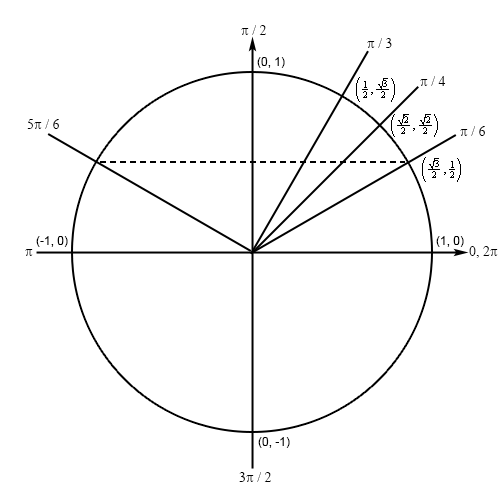Paul's Online Notes
Home / Calculus I / Review / Solving Trig Equations
Show Mobile Notice Show All Notes Hide All Notes
Mobile Notice
You appear to be on a device with a "narrow" screen width (i.e. you are probably on a mobile phone). Due to the nature of the mathematics on this site it is best views in landscape mode. If your device is not in landscape mode many of the equations will run off the side of your device (should be able to scroll to see them) and some of the menu items will be cut off due to the narrow screen width.

### Section 1.4 : Solving Trig Equations

1. Without using a calculator find all the solutions to $$4\sin \left( {3t} \right) = 2$$.

Show All Steps Hide All Steps

Hint : Isolate the sine (with a coefficient of one) on one side of the equation.
Start Solution

Isolating the sine (with a coefficient of one) on one side of the equation gives,

$\sin \left( {3t} \right) = \frac{1}{2}$
Hint : Use your knowledge of the unit circle to determine all the angles in the range $$\left[ {0,2\pi } \right]$$ for which sine will have this value.
Show Step 2

Because we’re dealing with sine in this problem and we know that the $$y$$-axis represents sine on a unit circle we’re looking for angles that will have a $$y$$ coordinate of $${\textstyle{1 \over 2}}$$. This means we’ll have an angle in the first quadrant and an angle in the second quadrant (that we can use the angle in the first quadrant to find). Here is a unit circle for this situation.Clearly the angle in the first quadrant is $$\frac{\pi }{6}$$ and by some basic symmetry we can see that the terminal line for the second angle must form an angle of $$\frac{\pi }{6}$$ with the negative $$x$$-axis as shown above and so it will be : $$\pi - \frac{\pi }{6} = \frac{{5\pi }}{6}$$.

Hint : Using the two angles above write down all the angles for which sine will have this value and use these to write down all the solutions to the equation.
Show Step 3

From the discussion in the notes for this section we know that once we have these two angles we can get all possible angles by simply adding “$$+ \,2\pi n$$ for $$n = 0, \pm 1, \pm 2, \ldots$$” onto each of these.

This then means that we must have,

$3t = \frac{\pi }{6} + 2\pi n \hspace{0.5in}{\rm{OR }}\hspace{0.5in}3t = \frac{{5\pi }}{6} + 2\pi n \hspace{0.5in} n = 0, \pm 1, \pm 2, \ldots$

Finally, to get all the solutions to the equation all we need to do is divide both sides by 3.

$\require{bbox} \bbox[2pt,border:1px solid black]{{t = \frac{\pi }{{18}} + \frac{{2\pi n}}{3} \hspace{0.5in} {\rm{OR }} \hspace{0.5in} t = \frac{{5\pi }}{{18}} + \frac{{2\pi n}}{3} \hspace{0.5in} n = 0, \pm 1, \pm 2, \ldots }}$